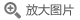## 经典力学的数学方法(第2版影印版)(英文版)

• 定价： ￥139
• ISBN：9787519255855
• 开 本：16开 平装
• 作者：(俄罗斯)V.I.阿诺...
• 立即节省：
• 2019-03-01 第1版
• 2019-03-01 第1次印刷### 导语### 内容提要这是莫斯科大学理论力学的优秀教材，论述了振动理论、刚体运动和哈密顿形式体系等动力学中的所有基本问题，特别强调了边分原理和分析力学及成为量子力学理论基石的哈密顿形式体系。在附录中介绍了经典力学与数学、物理学及其它领域的联系。可供理论力学专业、数学力学专业的研究生及科技人员参考。

### 目录

Preface
Preface to the second edition
Part I  NEWTONIAN  MECHANICS
Chapter 1  Experimental facts
1. The principles of relativity and determinacy
2. The galilean group and Newton's equations
3. Examples of mechanical systems
Chapter 2  Investigation of the equations of motion
4. Systems with one degree of freedom
5. Systems with two degrees of freedom
6. Conservative force fields
7. Angular momentum
8. Investigation of motion in a central field
9. The motion of a point in three-space
10. Motions of a system ofn points
11. The method of similarity
Part II  LAGRANGIAN MECHANICS
Chapter 3  Variational principles
12. Calculus of variations
13. Lagrange's equations
14. Legendre transformations
15. Hamilton's equations
16. Liouville's theorem
Chapter 4  Lagrangian mechanics on manifolds
17. Holonomic constraints
18. Differentiable manifolds
19. Lagrangian dynamical systems
20. E. Noether's theorem
21. D'Alembert's principle
Chapter 5  Oscillations
22. Linearization
23. Small oscillations
24. Behavior of characteristic frequencies
25. Parametric resonance
Chapter 6  Rigid bodies
26. Motion in a moving coordinate system
27. Inertial forces and the Coriolis force
28. Rigid bodies
29. Euler's equations. Poinsot's description of the motion
30. Lagrange's top
31. Sleeping tops and fast tops
Part III  HAMILTONIAN MECHANICS
Chapter 7  Differential forms
32. Exterior forms
33. Exterior multiplication
34. Differential forms
35. Integration of differential forms
36. Exterior differentiation
Chapter 8  Symplectic manifolds
37. Symplectic structures on manifolds
38. Hamiltonian phase flows and their integral invariants
39. The Lie algebra of vector fields
40. The Lie algebra of hamiltonian functions
41. Symplectic geometry
42. Parametric resonance in systems with many degrees of freedom
43. A symplectic atlas
Chapter 9  Canonical formalism
44. The integral invariant of Poincare-Cartan
45. Applications of the integral invariant of Poincare-Cartan
46. Huygens' principle
47. The Hamilton-Jacobi method for integrating Hamilton's canonical equations
48. Generating functions
Chapter 10  Introduction to perturbation theory
49. Integrable systems
50. Action-angle variables
51. Averaging
52. Averaging of perturbations
Appendix 1
Riemannian curvature
Appendix 2
Geodesics of left-invariant metrics on Lie groups and the hydrodynamics of ideal fluids
Appendix 3
Symplectic structures on algebraic manifolds
Appendix 4
Contact structures
Appendix 5
Dynamical systems with symmetries
Appendix 6
Appendix 7
Normal forms of hamiltonian systems near stationary points and closed trajectories
Appendix 8
Theory of perturbations of conditionally periodic motion, and Kolmogorov's theorem
Appendix 9
Poincare's geometric theorem, its generalizations and applications
Appendix 10
Multiplicities of characteristic frequencies, and ellipsoids
depending on parameters
Appendix 11
Short wave asymptotics
Appendix 12
Lagrangian singularities
Appendix 13
The Korteweg-de Vries equation
Appendix 14
Poisson structures
Appendix 15
On elliptic coordinates
Appendix 16
Singularities of ray systems
Index